Compressibility & Consolidation

# Compressibility & Consolidation - Notes | Study Civil Engineering SSC JE (Technical) - Civil Engineering (CE)

 1 Crore+ students have signed up on EduRev. Have you?

Compressibility And Consolidation

COEFFICIENT OF COMPRESSIBILITY (av)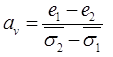e1 = Void Ratio at effective stress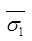e2 = Void ratio at effective stress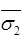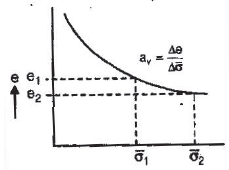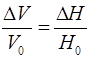ΔV = Change in volume in m3, or cm3.
V0 = Initial volume in m3 or cm3.
ΔH = Change in depth in ‘m’ or ‘cm’.
H0 = original depth in ‘m’ or ‘cm’.
Remember: Consolidation settlement is a function of effective stress and not the function of total stress.

COEFFICIENT OF COMPRESSION (Cc
(a)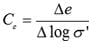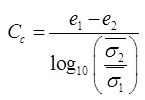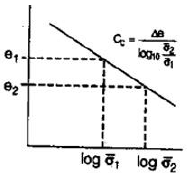(b) CC = 0.009 (WL- 10) For undisturbed clays of low to medium senstivity WL = liquid limit expressed in percent.

(c) CC = 0.007 ( WL- 7) For remoulded soil of low sensitivity

(d) CC = 0.40 (e0- 0.25) For undisturbed soil of medium sensitivity e0 = Initial void ratio

(e) For remoulded soil of low sensitivity.
CC =1.15(e0- 0.35)

(f) C =0.115 w where, w = Water content

OVER CONSOLIDATION RATIO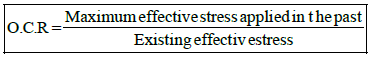O.C.R >1
For over consolidated soil.
O.C.R = 1
For normally consolidated soil.
O.C.R < 1
For under consolidated clay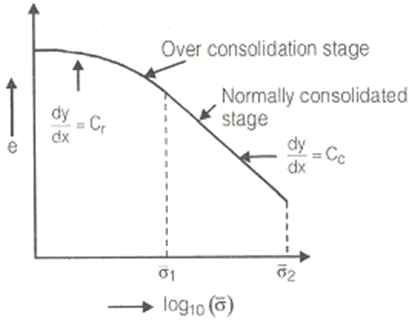DIFFERENTIAL EQUATION OF 1-D CONSOLIDATION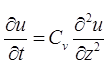where,
u = Excess pore pressure,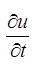= Rate of change of pore pressure
CV = Coefficient of consolidation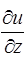= Rate of change of pore pressure with depth.

COEFFICIENT OF VOLUME COMPRESSIBILITY: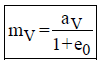where, e0 = Initial void ratio
mv = Coefficient of volume compressibility

COMPRESSION MODULUS: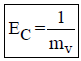where, Ec = Compression modulus.

DEGREE OF CONSOLIDATION
(i)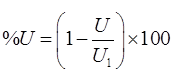where, % U = % degree of consolidation.
U = Excess pore pressure at any stage.
Ui =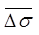= Initial excess pore pressureat
At t = 0, U = u1 ⇒ %u= 0%
at t = ∞ , u = 0 ⇒ %u =100%
(ii)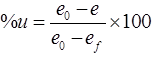where, ef = Void ratio at 100% consolidation
i.e., of t =∞
e = Void ratio at time ‘t’
e0= Initial void ratio i.e, at t = 0

(iii)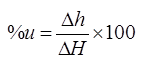where, ΔH = Final total settlement at the end of completion of primary consolidation i.e., at t =∞
Δh = Settlement occurred at any time ‘t’.

TIME FACTOR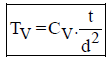where, Tv  = Time factor
CV = Coeff. of consolidation in cm2/sec.
d  = Length of drainage path
t  = Time in ‘sec’
d= Ho/2 For 2-way drainage d =H0 For one-way drainage.
where, H0 = Depth of soil sample.

(i)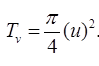, if u ≤ 60% T50 = 0.196

(ii)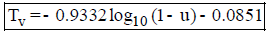, if u > 60%

METHOD TO FIND 'Cv
(i) Square Root of Time Fitting method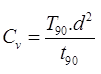where,T90 = Time factor at 90% consolidation
t90 = Time at 90% consolidation
d   = Length of drainage path.

(ii) Logarithm of Time Fitting method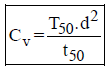where,T50 = Time factor of 50% consolidation
t50 = Time of 50% consolidation
Remember: Square root of  time fitting method is better for soil having higher secondary consolidation.

COMPRESSION RATIO:
(i) Initial compression Ratio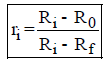where, Ri = Initial reading of dial gauge.
R0 = Reading of dial gauge at 0% consolidation
Rf = Final reading of dial gauge after secondary consolidation.

(ii) Primary Consolidation Ratio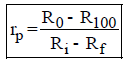where, R100 = Reading of dial gauge at 100% primary consolidation.

(iii) Secondary Consolidation Ratio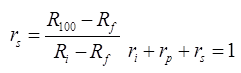TOTAL SETTLEMENT
S = Si + SP+Ss
where Si = Initial settlement, Sp=Primary Settlement Ss = Secondary settlement
(i) Initial Settlement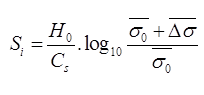For cohesionless soil.
where,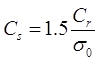where, Cr = Static one resistance in kN/m2
H0 = Depth of soil sample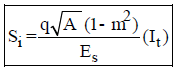for cohesive soil.
where, It = Shape factor or influence factor, A = Area.
Remember: For square footing , A = B2 and It = 1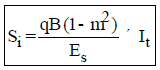-for strip footing
(ii) Primary Settlement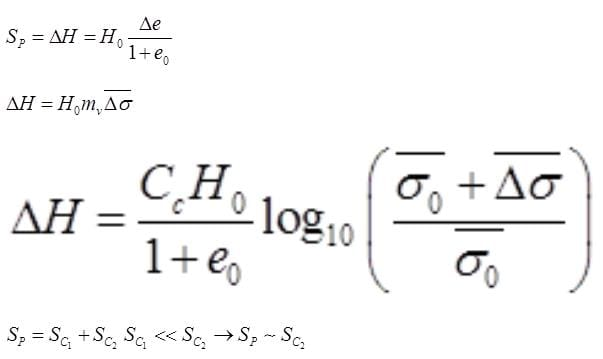sc1 = Settlement for over consolidated stage
sc1 = Settlement normally consolidated stage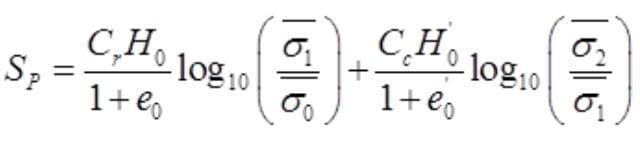(iii) Secondary Settlement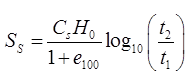where, H0 ~ H100
H100 = Thickness of soil after 100% primary consolidation.
e100 = Void ratio after 100% primary consolidation.
t2  = Average time after t1 in which secondary consolidation is calculated.

The document Compressibility & Consolidation - Notes | Study Civil Engineering SSC JE (Technical) - Civil Engineering (CE) is a part of the Civil Engineering (CE) Course Civil Engineering SSC JE (Technical).
All you need of Civil Engineering (CE) at this link: Civil Engineering (CE)

## Civil Engineering SSC JE (Technical)

2 videos|122 docs|50 tests
 Use Code STAYHOME200 and get INR 200 additional OFF

## Civil Engineering SSC JE (Technical)

2 videos|122 docs|50 tests

Track your progress, build streaks, highlight & save important lessons and more!

,

,

,

,

,

,

,

,

,

,

,

,

,

,

,

,

,

,

,

,

,

;